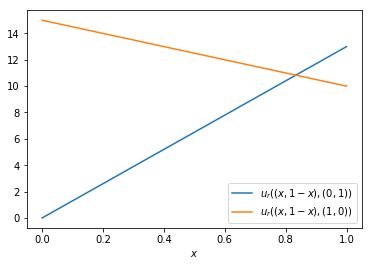# Nash equilibria - Solutions¶

1. Give the definition of a best response.

2. For the following games identify the best reponses:

1. $A = \begin{pmatrix} \underline{2} & \underline{1}\\ 1 & \underline{1}\end{pmatrix} \qquad B = \begin{pmatrix} \underline{1} & \underline{1}\\ 1 & \underline{3}\end{pmatrix}$

2. $A = \begin{pmatrix} 2 & 1 & 3 & 17\\ \underline{27} & 3 & 1 & 1\\ 4 & \underline{6} & \underline{7} & \underline{18} \end{pmatrix} \qquad B = \begin{pmatrix} 11 & 9 & 10 & \underline{22}\\ 0 & \underline{1} & \underline{1} & 0\\ 2 & 10 & \underline{12} & 0 \end{pmatrix}$

3. $A = \begin{pmatrix} \underline{3} & \underline{3} & 2 \\ 2 & 1 & \underline{3} \end{pmatrix} \qquad B = \begin{pmatrix} 2 & 1 & \underline{3} \\ 2 & \underline{3} & 2 \end{pmatrix}$

4. $A = \begin{pmatrix} \underline{3} & -1\\ 2 & \underline{7}\end{pmatrix} \qquad B = \begin{pmatrix} -3 & \underline{1}\\ \underline{1} & -6\end{pmatrix}$

3. Represent the following game in normal form:

Assume two neighbouring countries have at their disposal very destructive armies. If both countries attack each other the countries' civilian population will suffer 10 thousand casualties. If one country attacks whilst the other remains peaceful, the peaceful country will lose 15 thousand casualties but would also retaliate causing the offensive country 13 thousand casualties. If both countries remain peaceful then there are no casualties.

1. Clearly state the players and strategy sets.

Players: $\{P_1, P_2\}$. The strategy space is $\\{A,P\\}$. We take the utility to be the number of casualties suffered (players aim to minimize their utility):

$$A=\begin{pmatrix} 10&13\\ 15&0 \end{pmatrix} \qquad B=\begin{pmatrix} 10&15\\ 13&0 \end{pmatrix}$$

2. Plot the utilities to both countries assuming that they play a mixed strategy while the other country remains peaceful.

Plot of (see below)

$$u_r((x,1-x),(0,1))=13x$$

(Same plot for $P_2$)

3. Plot the utilities to both countries assuming that they play a mixed strategy while the other country attacks.

Plot of (see below):

$$u_r((x,1-x),(1,0))=10x+15(1-x)=15-5x$$

(Same plot for $P_2$)

4. Obtain the best responses of each player.

The pure best responses are obtained:

$$A=\begin{pmatrix} \underline{10}&13\\ 15&\underline{0} \end{pmatrix} \qquad B=\begin{pmatrix} \underline{10}&15\\ 13&\underline{0} \end{pmatrix}$$

by finding the intersection of the following plots:

$$10y+13(1-y)=15y\Rightarrow y=13/18$$

Thus the full best response is:

$$\sigma^*=\begin{cases} (1, 0),&y>13/18\\ (0, 1),&y<13/18\\ \text{indifferent},&y=13/18 \end{cases}$$

4. State and prove the best response condition.

5. Define Nash equilibria.

In :
%matplotlib inline
import matplotlib.pyplot as plt
import numpy as np
xs = np.linspace(0, 1, 500)
utility_against_peaceful = [13 *  x for x in xs]
utility_against_attack = [15 - 5 * x for x in xs]
plt.plot(xs, utility_against_peaceful, label="$u_r((x,1-x),(0,1))$")
plt.plot(xs, utility_against_attack, label="$u_r((x,1-x),(1,0))$")
plt.xlabel("$x$")
plt.legend();Previous

Next